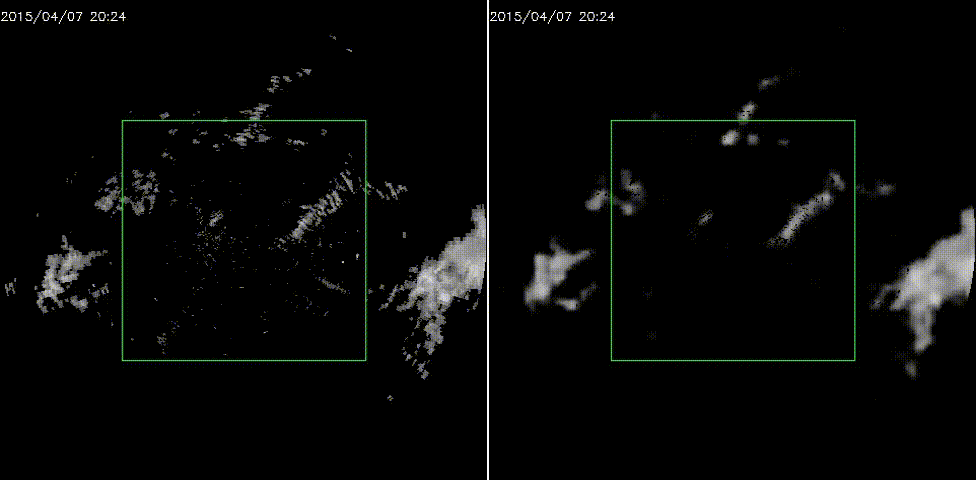### 数据集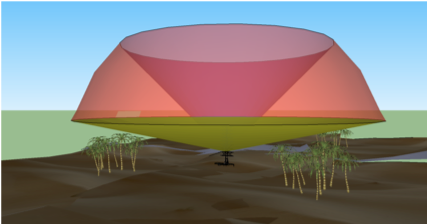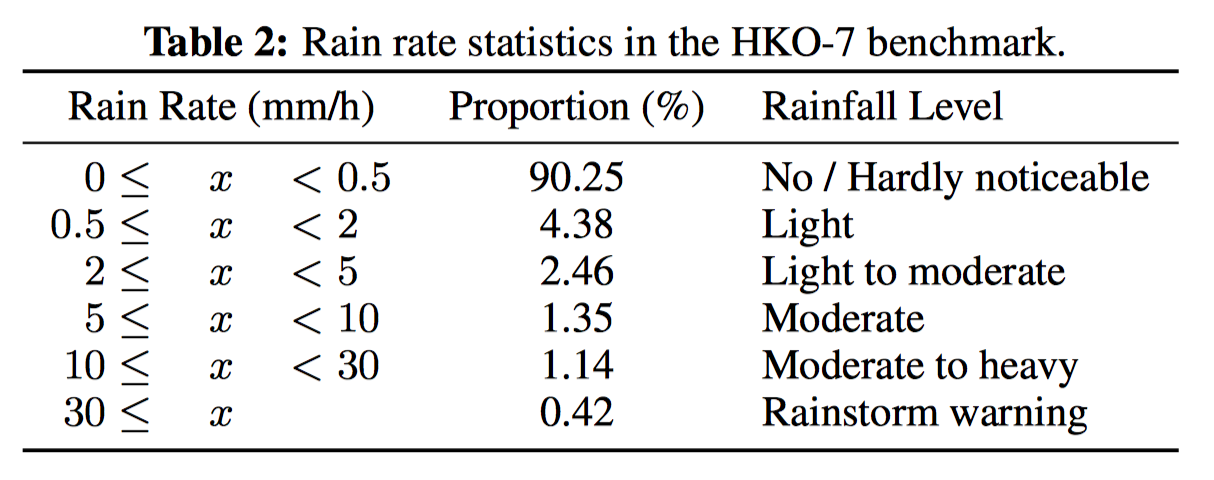$$w ( x ) = \left\{ \begin{array} { l l } { 1 , } & { x < 2 } \\ { 2 , } & { 2 \leq x < 5 } \\ { 5 , } & { 5 \leq x < 10 } \\ { 10 , } & { 10 \leq x < 30 } \\ { 30 , } & { x \geq 30 } \end{array} \right.$$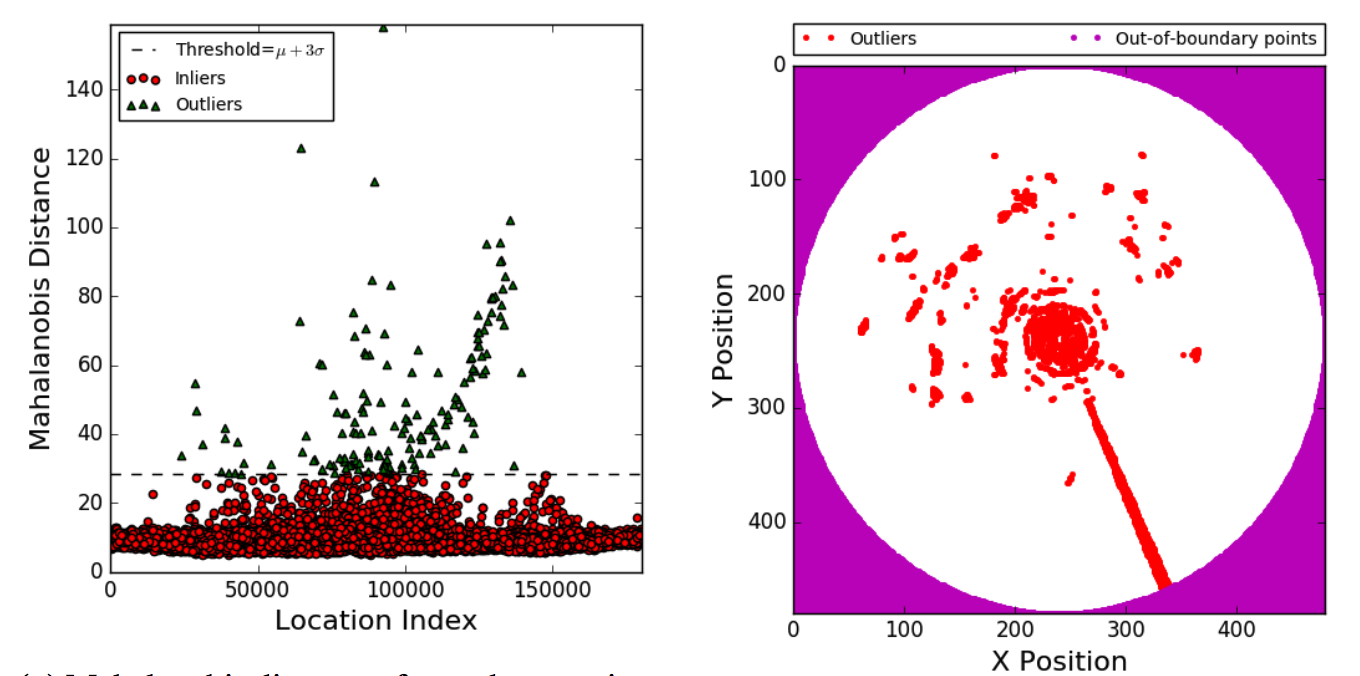### ConvLSTM

ConvLSTM 使用卷积替换 FC-LSTM，利用卷积操作提取空间信息，FC-LSTM 和 ConvLSTM 的区别主要在输入 $\mathcal { X } _ { 1 } , \ldots , \mathcal { X } _ { t }$，候选态 $\mathcal { C } _ { 1 } , \ldots , \mathcal { C } _ { t }$，隐藏态 $\mathcal { H } _ { 1 } , \ldots , \mathcal { H } _ { t }$ 和三个门 $i _ { t } , f _ { t } , o _ { t }$ 都是三维张量，其中最后两个维度是 spatial 空间维度。如下图：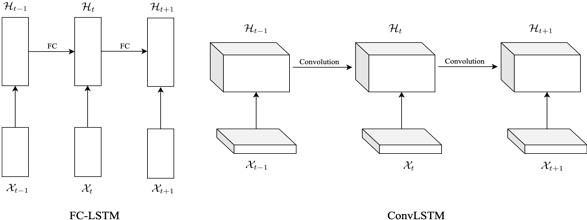ConvLSTM 的公式:

\begin{equation} \begin{aligned} i _ { t } &= \sigma \left( W _ { x i } * \mathrm { X } _ { t } + W _ { h i } * \mathrm { H } _ { t - 1 } + W _ { c i } \circ \mathrm { C } _ { t - 1 } + b _ { i } \right)\\ f _ { t } &= \sigma \left( W _ { x f } * \mathrm { X } _ { t } + W _ { h f } * \mathrm { H } _ { t - 1 } + W _ { c f } \circ \mathrm { C } _ { i - 1 } + b _ { f } \right)\\ C _ { t } &= f _ { t } \circ C _ { t - 1 } + i _ { t } \circ \tanh \left( W _ { x c } * X _ { t } + W _ { h c } * H _ { t - 1 } + b _ { c } \right)\\ o _ { t } &= \sigma \left( W _ { x o } * \mathrm { X } _ { t } + W _ { h o } * \mathrm { H } _ { t - 1 } + W _ { c o } \circ \mathrm { C } _ { t } + b _ { o } \right)\\ \mathrm { H } _ { t } &= o _ { t } \circ \tanh \left( \mathrm { C } _ { t } \right) \end{aligned} \end{equation}

$*$ 是卷积操作，$\circ$ 是哈达玛乘积。因此 FC-LSTM 与 ConvLSTM 的主要差别在于将矩阵乘法替换成了卷积操作，即 input-to-state 和 state-to-state 的转换中使用的是卷积而不是全连接。

If we view the states as the hidden representations of moving objects, a ConvLSTM with a larger transitional kernel should be able to capture faster motions while one with a smaller kernel can capture slower motions. Also, if we adopt a similar view as , the inputs, cell outputs and hidden states of the traditional FC-LSTM represented by (2) may also be seen as 3D tensors with the last two dimensions being 1. In this sense, FC-LSTM is actually a special case of ConvLSTM with all features standing on a single cell.

Pytorch 实现可以参考 ConvLSTM，我合并了八次卷积操作，所以整个计算量和普通的卷积差不多，偏置项影响不大。

### TrajGRU

TrajGRU(Trajectory GRU) 来自于施行健的第二篇文章 Deep Learning for Precipitation Nowcasting: A Benchmark and A New Model ，在这篇文章中，作者提出了一个新的 Benchmark 也就是上面介绍的数据集，对比了 ConvGRU、不使用/使用加权损失函数训练、Conv2d、Conv3d 等几种方法，构建了一个 Encoder-Forecaster 模型。

ConvGRU 类似于上面介绍的 ConvLSTM，只是将 LSTM 替换成了 GRU。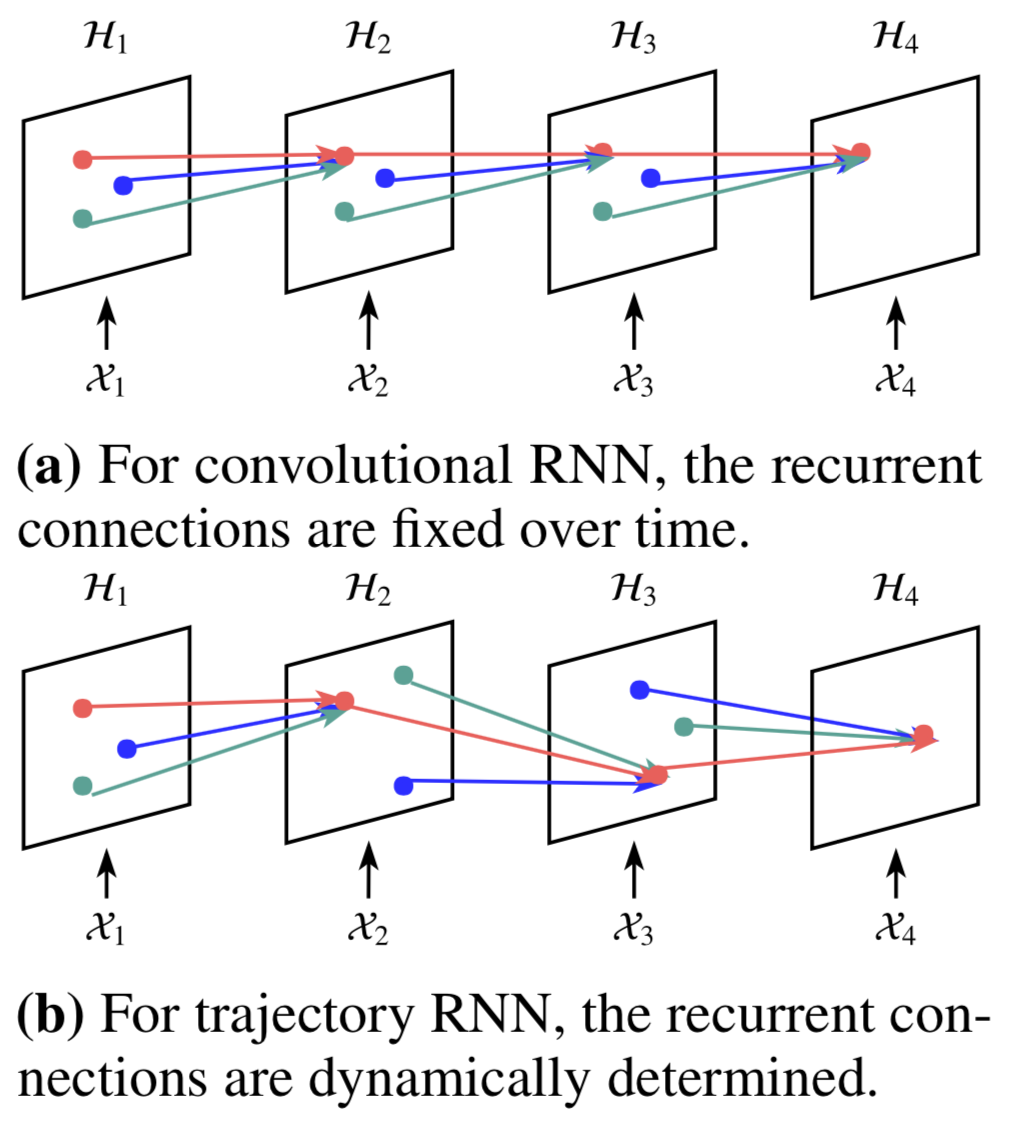TrajGRU 的公式如下，首先使用一个子网络提取光流 $\mathcal{U}_t,\mathcal{V}_t$，然后使用 wrap 函数变换，有 $L$ 个链接。

\begin{align} {{\mathcal{U}}_{t}},{{\mathcal{V}}_{t}} &=\gamma \left( {{\mathcal{X}}_{t}},{{\mathcal{H}}_{t-1}} \right) \\ {{\mathcal{Z}}_{t}} &=\sigma \left( {{\mathcal{W}}_{xz}}*{{\mathcal{X}}_{t}}+\sum\limits_{l=1}^{L}{\mathcal{W}_{hz}^{l}}* warp \left( {{\mathcal{H}}_{t-1}},{{\mathcal{U}}_{t,l}},{{\mathcal{V}}_{t,l}} \right) \right) \\ {{\mathcal{R}}_{t}} &=\sigma \left( {{\mathcal{W}}_{xr}}*{{\mathcal{X}}_{t}}+\sum\limits_{l=1}^{L}{\mathcal{W}_{hr}^{l}}* warp \left( {{\mathcal{H}}_{t-1}},{{\mathcal{U}}_{t,l}},{{\mathcal{V}}_{t,l}} \right) \right) \\ \mathcal{H}_{t}^{\prime } &=f\left( {{\mathcal{W}}_{xh}}*{{\mathcal{X}}_{t}}+{{\mathcal{R}}_{t}}{}^\circ \left( \sum\limits_{l=1}^{L}{\mathcal{W}_{hh}^{l}}* warp \left( {{\mathcal{H}}_{t-1}},{{\mathcal{U}}_{t,l}},{{\mathcal{V}}_{t,l}} \right) \right) \right) \\ {{\mathcal{H}}_{t}} &=\left( 1-{{\mathcal{Z}}_{t}} \right){}^\circ \mathcal{H}_{t}^{\prime }+{{\mathcal{Z}}_{t}}{}^\circ {{\mathcal{H}}_{t-1}} \end{align}

wrap 函数的 mxnet 可以参考 traj_rnn.py#L14，pytorch 没有该函数，可以参考我的实现 trajGRU.py#L9

### Encoder-Forecaster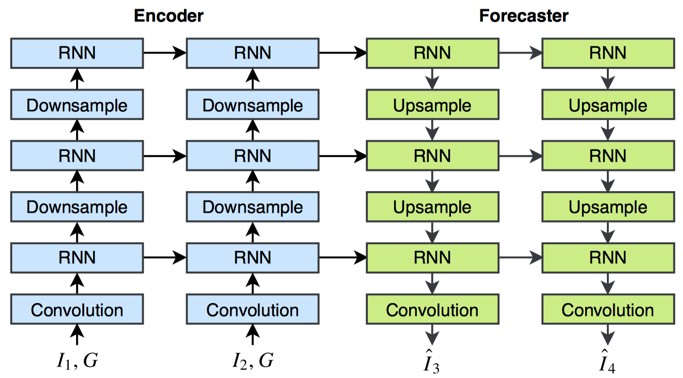Encoder 的初始隐藏态和 Forecaster 的初始输入为 0，因为这两个没有输入。最后的输出则通过一个 $1\times 1$ 的卷积层进行回归。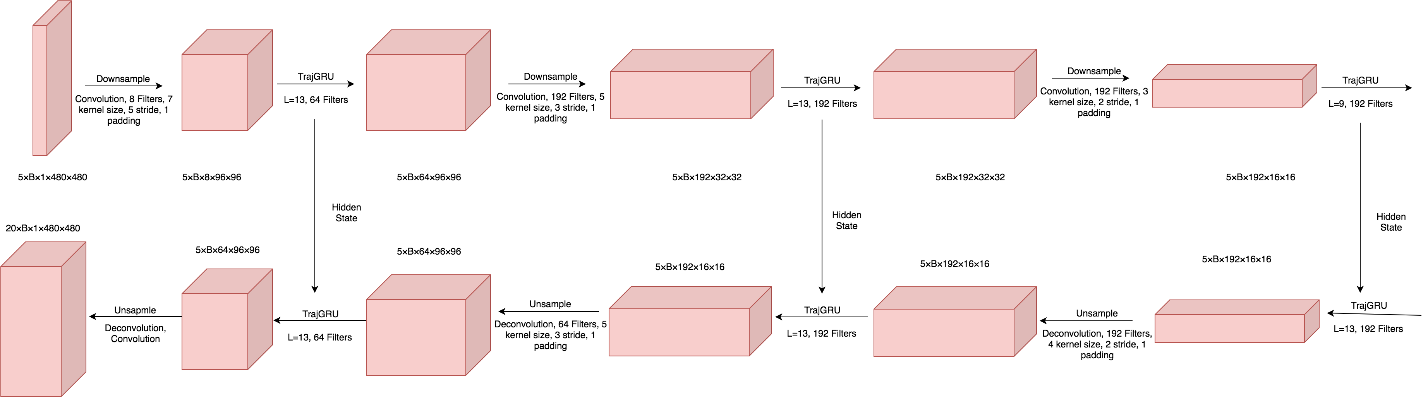### 总结